# GMAT Math : DSQ: Calculating the height of a right triangle

## Example Questions

### Example Question #62 : Right Triangles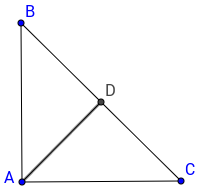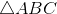is a right triangle where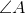is a right angle. What is the length of the height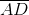?

(1)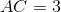(1)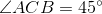Statement 2 alone is sufficient

Both statements together are sufficient

Each statement alone is sufficient

Statement 1 alone is sufficient

Statements 1 and 2 together are not sufficient.

Both statements together are sufficient

Explanation:

To know the length of the height triangle, we would need to know the lengths of the triangle or the angles to have more information about the triangle.

Statement 1 only gives us a length of a side. There is nothing more we can calculate from what we know so far.

Statement 2 alone tells us that the triangle is isoceles. Indeed, ABC is a right triangle, if one of its angle is 45 degrees, than so must be another. Now, we are able to tell that the length of the height would be the same as half the hypothenuse. A single side would be sufficient to answer the problem. Statment 1 gives us that information. Therefore, both statements together are sufficient.

### Example Question #63 : Right Triangles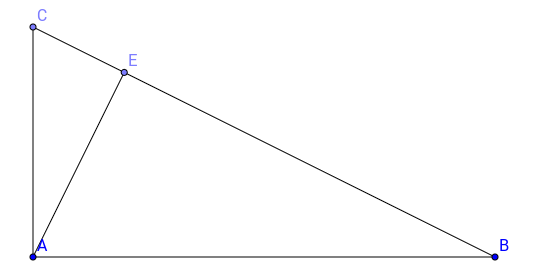What is the length of the height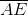of right triangle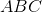, whereis a right angle?

(1)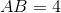(2)Each statement alone is sufficient

Both statements together are sufficient

Statement (1) alone is sufficient

Statements (1) and (2) together are not sufficient.

Statement (2) alone is sufficient

Both statements together are sufficient

Explanation:

Since we are told that triangle ABC is a right triangle, to find the height, we just need the length of at least 2 other sides. From there, we can find the length of the height since in a right triangle, the height divides the triangle into two triangles with the same proportions. In other words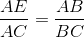. Therefore, we need to know the length of the sides of the triangle.

### Example Question #64 : Right Triangles

Consider right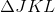.

I) The longest side,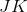, has a length ofmeters.

II)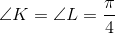.

What is the height of?

Both statements are needed to answer the question.

Statement II is sufficient to answer the question, but statement I is not sufficient to answer the question.

Either statement is sufficient to answer the question.

Statement I is sufficient to answer the question, but statement II is not sufficient to answer the question.

Both statements are needed to answer the question.

Explanation:

The height of a right triangle will be one of its side lengths.

I) tells us the length of our hypotenuse.

II) gives us the other two angle measurements.

They are both 45 degrees, which makes JKL a 45/45/90 triangle with side length ratios of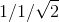.

Which we can use to find the height.

### Example Question #65 : Right Triangles

What is the height of the right triangle?

1. The area of the right triangle is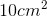.
2. The base of the right triangle measures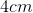.

Statement 1 alone is sufficient, but statement 2 alone is not sufficient to answer the question.

Both statements taken together are sufficient to answer the question, but neither statement alone is sufficient.

Statements 1 and 2 are not sufficient, and additional data is needed to answer the question.

Each statement alone is sufficient to answer the question.

Statement 2 alone is sufficient, but statement 1 alone is not sufficient to answer the question.

Both statements taken together are sufficient to answer the question, but neither statement alone is sufficient.

Explanation:

Statement 1: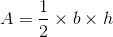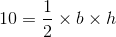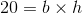More information is required to answer the question because our base and height can beandorandStatement 2: We're given the base so we can narrow down the information from Statement 1 toand. If the base is, then the height must beBoth statements taken together are sufficient to answer the question, but neither statement alone is sufficient.

### Example Question #66 : Right Triangles

What is the height of the rigth triangle?

1. The area of the right triangle is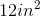.
2. The perimeter of the right triangle is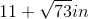Both statements taken together are sufficient to answer the question, but neither statement alone is sufficient.

Each statement alone is sufficient to answer the question.

Statement 1 alone is sufficient, but statement 2 alone is not sufficient to answer the question.

Statements 1 and 2 are not sufficient, and additional data is needed to answer the question.

Statement 2 alone is sufficient, but statement 1 alone is not sufficient to answer the question.

Statements 1 and 2 are not sufficient, and additional data is needed to answer the question.

Explanation:

Statement 1: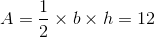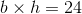Additional information is required because our base and height can beandand, orandStatement 2: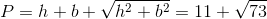Even if we solve for our two values, we will not be able to determine which is the base and which is the height.

Statements 1 and 2 are not sufficient, and additional data is needed to answer the question.

Tired of practice problems?

Try live online GMAT Math prep today.